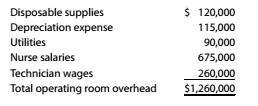Chapter 10, Problem 10.16E

Chapter
Section
Textbook Problem

Predetermined factory overhead rate Mt Ellis Medical Center has a single operating room that is used by local physicians to perform surgical procedures. The cost of using the operating room is accumulated by each patient procedure and includes the direct materials costs (drugs and medical devices), physician surgical time, and operating room overhead. On March 1 of the current year, the annual operating room overhead is estimated to be:The overhead costs will be assigned to procedures based on the number of surgical room hours. Mt Ellis Medical Center expects to use the operating room an average of 12 hours per day, seven days per week. In addition, the operating room will be shut down two weeks per year for general and maintenance repairs. a. Determine the predetermined operating room overhead rate for the year. b.Shirlee Greer had a 3.4-hour procedure on March 18. How much operating room overhead would be charged to her procedure, using the rate determined in part (a)? c. During March, the operating room was used 330 hours. The actual overhead costs incurred for March were $101,750. Determine the overhead under- or overapplied for the period. To determine (a) Concept Introduction: Overhead: This cost is incurred at the time of production of manufaturing units. This is an indirect cost. Factory Overhead rate: - It is the rate on the basis of which factory overhead cost is allocated to the units. It can be computed by dividing the total factory overhead incurred by a number of units produce. To compute: The predetermined operating room overhead rate for the year. Explanation As given in the question, total operating room overhead is$1,260,000.

Now, computation of number of operating room hour is:

Annual operating room hour=(hour per day×days per week×weeks per year)=(12×7×50)=

To determine

(b)

Concept Introduction:

This cost is incurred at the time of production of manufaturing units. This is an indirect cost.

- it is the rate on the basis of which factory overhead cost is allocated to the units. It can be computed by dividing the total factory overhead incurred by a number of units produce.

To compute:

The amount of overhead.

To determine

(c)

Concept Introduction:

This cost is incurred at the time of production of manufaturing units. This is an indirect cost.

- it is the rate on the basis of which factory overhead cost is allocated to the units. It can be computed by dividing the total factory overhead incurred by a number of units produce.

To calculate:

The under applied or over applied overhead.

Still sussing out bartleby?

Check out a sample textbook solution.

See a sample solution

The Solution to Your Study Problems

Bartleby provides explanations to thousands of textbook problems written by our experts, many with advanced degrees!

Get Started

Find more solutions based on key concepts